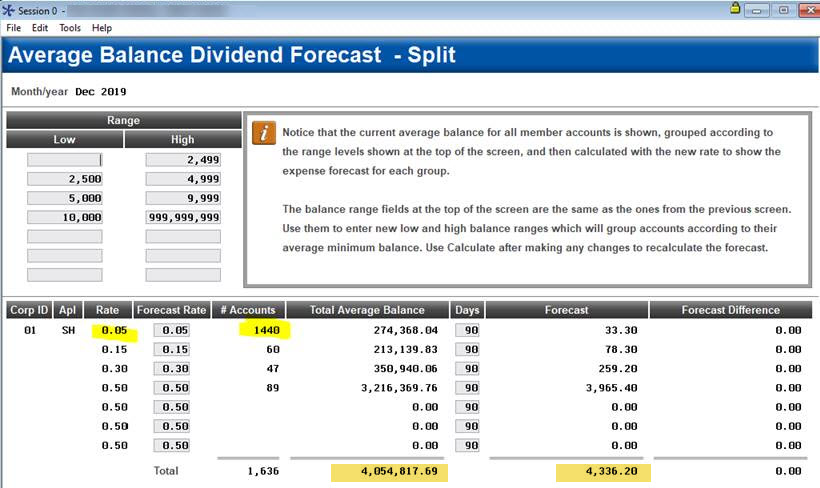If your regular share account is set up with a split rate, assuming the majority of your accounts are at the base rate, most credit unions elect to use that rate as the average rate on the call report.  The call report is looking for an average rate, not a weighted one.

If you wanted to calculate the weighted average rate on a split-rate share product for other reasons, you could use Tool #344 Div/Int Split Rate Forecasting.  Take the Total Forecasted Dividends, divide by the Total Average Balance, and multiply by 100.

In the sample below, the calculation would be:  ( \$4,336.20 / \$4,054,817.69 ) * 100 = 0.10693%
Therefore this dividend application has a weighted average rate of 0.106%.